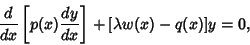## Sturm-Liouville Equation

A second-order Ordinary Differential Equationwhereis a constant andis a known function called either the density or Weighting Function. The solutions (with appropriate boundary conditions) ofare called Eigenvalues and the correspondingEigenfunctions. The solutions of this equation satisfy important mathematical properties under appropriate boundary conditions (Arfken 1985).

Arfken, G. Sturm-Liouville Theory--Orthogonal Functions.'' Ch. 9 in Mathematical Methods for Physicists, 3rd ed. Orlando, FL: Academic Press, pp. 497-538, 1985.